অসমীয়া   বাংলা   बोड़ो   डोगरी   ગુજરાતી   ಕನ್ನಡ   كأشُر   कोंकणी   संथाली   মনিপুরি   नेपाली   ଓରିୟା   ਪੰਜਾਬੀ   संस्कृत   தமிழ்  తెలుగు   ردو

# Brain Teasers- Riddles for kids

### Riddles for kids

#### Why do copper become shinier after being soaked in a fruit juices like grapefruit or lime?

Copper is very slowly oxidized on the surface in moist air, giving a green or dull coating or verdigris. This is basic copper carbonate CuCO3. Cu(OH)2 and is familiar on the roofs of buildings covered with copper sheet, and also on copper statues. The acids in the fruit juices react with the basic coating and bring out the original luster of copper.

#### Why does an oil film floating on water appear coloured?

The colours in soap or oil film are due to thin film interference. Birefly, light reflected from the first surface of the film interferes with the light reflected from the second surface. Whether the interference is constructive or destructive depends on the wavelength of the light, the refractive index of the film, and the path length of the light inside the film. For further information,

When colored lights are mixed together, a white colour is formed. When pigment colours are mixed together the colour formed is black. Why?

The red, blue and green coloured light of the spectrum are called the primary colours in light. We can form all coloured lights by blending these primary colours from the spectrum. Primary colours of light-red, blue and green can be mixed to make white light. These primary colours of light are used in creating colour television. The principle of forming colours by combining parts of the spectrum is called additive colour mixture of colour by addition. This applies only with colours in light.

The other basic way to mix colour is called subtractive colour mixture or colour by subtraction. This applies only with colours of pigment. When the spectrum falls on a coloured surface due to the presence pigment, we do not see the whole spectrum; we see only some parts of it. The pigment absorbs parts of the light spectrum. In other words, parts of it. The pigment absorbs parts of the light spectrum. In other words, parts of the spectrum of white light are removed. This process, colour by subtraction, forms the closest link between colour in light and colour in pigment. The magenta, yellow and cyan are called primary colours of pigment. They can be mixed in various proportions to form pigments of different colours. When magenta, yellow and cyan pigments are mixed, colours of the whole spectrum are absorbed (or removed). As a result, black is obtained.

For further information on colour mixing, please refer to the URLs below:

#### How does temperature and dilution affect a solution’s buffering capacity?

A buffer solution is one that resists changes in pH when small quantities of an acid or an alkali are added to it. Most buffer solutions are made up using a weak acid and its sodium salt. Since acids react with bases, while bases react with acids, an acid-base buffer solution must contain reasonable amounts of both a weak acid and a weak base. It has been found that an acid –base buffer solution is most effective when it contains both a weak acid and the conjugate base of that weak acid in comparable concentrations. By comparable concentrations we mean that the two concentrations should not differ by more than about one order of magnitude. The pH of an aqueous acid-base buffer solution can be calculated directly from the equation for the ionization constant of the weak acid. Both the concentration of the weak acid and the concentration of its conjugate base must be known.

A typical lab buffer is acetic acid CH3COOH and its salt CH3COONa. The ionization constant for CH3COOh is:

Since buffer solutions only involve weak acids and bases, it can be assumed that ionization of the acetic acid and hydrolysis of the acetate ions are negligible. Therefore, when the initial concentrations of the acid and conjugate base are the same, the pH of the buffer is equal to the pka of the acid. After the addition of HCI, Complete ionization of HCI occurs: Neutralization of the HCI by acetate ions occurs: the used up hydrogen ions change the number of moles of acetic acid and acetate ions, and hydrogen ions:

Moles of CH3COO- =initial moles of CH3COO- -initial moles of HCI Moles of CH3COOH = initial moles of CH3COOH + initial moles of HCI Now the formula for ionization constant can be used to determine the new [H+] and the new pH.

Since temperature affects the dissociation of some weak acids and bases, the ka value of buffers is temperature dependent. With a 2-fold dilution, the value of ka will remain constant while the [H+] will reduce to half for a monobasic salt like CH3COONa. Hence, the pH value increases by log 2.

#### Have 4 from 5 and 3

Your mother asks you to bring 4 litres of water from the nearest pond and you are given only jugs.On jug has a content of 3 litres, and the other one has a content 0f 5 litres. How do you bring 4 litres of water?

Solution

• Fill the 5 litre jug.
• Then fill the 3 litre jug to the top with water from the 5 litre jug.
• Now you have 2 litres of water in the 5 litre jug.
• Dump out the 3 litre jug back to the pond
• and pour what’s in the 5 litre jug into the 3 litre jug.
• Then refill the 5 litre jug, and fill up the 3 litre jug to the top.

Since there were already 2 litres of water in the 3 litre jug, 1 litre is removed from the5 litre jug,Leaving 4 litres of water in the 5 litre jug.

#### Puzzle 1

We have 12 coins and a balance. 11 coins are of the same weight, but one coin differs in weight. You may perform three weighings to find out which coin has a different weight. Note that you don’t know whether the coin with different weight is heavier or lighter. How should you do these three experiments to find out?

Solution:

Unfortunately, this solution requires an extensive. Divide the 12 coins in three groups of four coins each: A, B and C. then start weighing group A against B. now the following two situations are possible:

(1) The A against B weighing is equal: This means that one of the coins in group C has a different weight. Now take two of the coins in group C (C1 and C2) and weigh them against two coins of group A (A1 and A2, of which you know they have a correct weight). Again, there are two possible results:

(1a) C1 + C2 are as heavy as A1+A2: This means that C3 or C4 is the coin with a different weight, now you can determine which one of it is by weighing for instance C3 against A1 (=a correct coin).

(1b) C1+C have different weight than A1 +A2: This means that C1 or C is the coin with a different weight, so now you can determine which one it is by weighing for instance C1 against A1 (= a correct coin).

(2) A and B have different weights: Call the lightest group A and the heaviest group B, Now you know that the remaining group C consists of all correct coins. Then do the following weighing: take two coins from group A and two coins from group B ( A1, A2, B1, and B2) and weigh them against one coin from A, one coin from B and two coins from C (A3, B3, C1, and C2). Now the following three situations are possible:

(2a) A1 + A2 + B1 + B2 are as heavy as A3 + B3 + C1 + C2: This means that A4 or B4 has a different weight, now you can determine which one it is by weighing for instance A4 against C1 ( =a correct coin).

(2b) A1 + A2 + B1 + B2 are lighter than A3 + B3 + C1 + C2: This means that either A1 or A2 are of different weight (lighter) or B3 is of different weight (heavier). Now weigh A1 + B3 against C1 + C2 which can result in the following situations:

(2bi) A1 + B3 are as heavy as C1 + C2:
Which means that A2 has a different weight.

(2bii) A1 + B3 are lighter than C1 + C2:
Which means that A1 has a different weight.

(2biii) A1 + B3 are heavier than C1 + C2:
Which means that B3 has a different weight.

(2c) A1 + A2 + B1 + B2 are heavier than A3 + B3 + C1 + C2: similar explanation as in situation 2b.

#### Puzzle 2

A swimmer jumps from a bridge over a canal and swims 1 kilometer stream up. After that first kilometer, he passes a floating cork. He continues swimming for half an hour and then turns around and swims back to the bridge. The swimmer and the cork arrive at the bridge at the same time. The swimmer has been swimming with constant speed.

#### How fast does the water in the canal flow?

Solution: If you have written down a full paper of mathematical formulae, you have been thinking too hard… It is obvious that the cork does not move relatively to the water (i.e. has the same speed as the water). So if the swimmer is swimming away from the cork for half an hour (up stream), it will take him another half hours to swim back to the cork again (down stream, where the reaches the cork at the moment it is passing the bridge). So in that one hour time, the cork has floated from 1 kilometer up stream to the bridge. Conclusion: The water in the canal flows at a speed of 1 km/h.

#### Puzzle 3

Four men want to crass a bridge. They all begin on the some side. It is night, and they have only one flashlight with them. At most two men can cross the bridge at a time, and any party crosses, either one of two people must have the flashlight with them. Them flashlight must be walked back and forth, it cannot be thrown back, etc. each man walks at a different speed. A pair must walk together at the speed of the slower man. Man
1 needs 1 minute to cross the bridge, man 2 needs 2 minutes, man 3 needs 5 minutes and man 4 needs 10 minutes. For example, if man 1 and man 3 walk across together, they need 5 minutes. What is the shortest time that is needed for all four to cross the bridge?

Solution: Answer: 17 minutes, first, man 1 and man 2 walk across the bridge. This takes 2 minutes. After this, man 1 walks back with the flashlight. This takes 1 minute. Then man 3 and man 4 walk across the bridge. This takes 10 minutes. After this, man 2 walks back with the flashlight. This takes 2 minutes. Then man 1 and 2 walk across the bridge. This takes 2 minutes as before. In total: 2 + 1 + 10 + 2 + 2 = 17 minutes.

#### How they divide the work so that it is done in the shortest possible time

Asha comes to help Lata do some work. While Asha can do the work in two hours, Lata would have taken three hours. How they divide the work so that it is done in the shortest possible time?

The simplest way to figure this out is realise that the shortest time taken is when they finish together. Clearly, the piece of work must be divided so that Asha is given more work than Lata , since she is 1.5 times faster than Lata. Another way of seeing it is, their speed is in a ratio of 3:2.so if they divide the work in this ratio, that is, if Asha does three times as much work as Lata, they will finish together. So Asha must do 3/5 of the work. Since she takes 2 hours to do the entire piece of work, she will take (3/5) × 2 = 1.2hrs = 1 hour, 12 minutes to do her share. You can check that Lata can do her 2/5 Share of the work in the same time. This is the shortest time in which they can complete the work.

#### How far away from Madras will we meet?

I leave from Madras to Vellore, 150 km away, at 10: AM. My car is old and warms up slowly. So it travels for the first one hour at 10 km/hr, for the next hour at 20 km/hr, the next 30 km/hr, etc., increasing its speed by 10 km/hr at the end of every hour. My brother leaves from Vellore towards Madras at the same time by his new super fast car. Since it is very fast, he starts at 50 km/hr and slows down by 10 km/hr every hour. At what time will we meet on the road? How far away from Madras will we meet?

I take 5 hours to do the trip, and travel the last 50 km at 50 km/hr. So my brother makes exactly the reverse of my trip in terms of speed. Hence, we will meet exactly after half the time, that is, after 2.5 hrs. At this point, I have traveled 10 km at 10 km/hr, another 20 km at 20 km/hr and 15 km at 30 km /hr. So we meet 45 km from Madras at 12:30.

#### Chess Number Game

Europeans and Indians participated in a chess tournament. Each contestant played each other contestant once. There were ten times as many Indians as Europeans. But they only won four-and-a-half times as many points as the Europeans. How many Europeans were there in the tournament and how many points did they get. (1 point for a win, ½ for a draw. No points if your lose)

1. Let N be the number of Europeans. Then, number of Indians = 10N

The Europeans play N (N-1)/2 games among themselves and 10N2 games against the Indians.
The MOST points the Europeans can get is therefore N(N-1)/2 + 10N2 (which is achieved when they win all their games with the Indians)
The Indians play 10N (10N-1)/2 among each other. This represents the LEAST number of points they can score (assuming they lose all their games to the Europeans)
This means….

4.5 MAX EUROPEAN PTS ≥ MIN INDIAN PTS
or 4.5x(N(N-1)/2+10 N2)≥10(10N-1)/2 which simplifies to
5.5≥5.5N
This inequality does hold for N>1 and the equality holds only for N=1 therefore there is only 1 European.
The European beats all the Indians to get 10points. The Indians score 10x9/2 points in the games among themselves for a total of 45 points.

#### Can you make this correct by moving only one number?

Q.2: What given below is, of course, a wrong equation?
62-63=1
Can you make this correct by moving only one number?!
(you can take any one of the above numbers and move to any other position so that the equation them becomes correct!)

1. Move the first 6 onto the 2 as a power to make 64(26=64).

26-63=1

#### How many seconds would it take Rajesh to reach the top?

Rajesh takes the underground train to work and uses an escalator at the railway station. While the escalator is going up Rajesh runs up 8 steps of the escalator. It takes him 37.5 seconds to reach the top of the escalator. If he runs up 13 steps of the escalator, then it takes him only 30.0 seconds to reach the top. How many seconds would it take Rajesh to reach the top if he did not run up any steps of the escalator at all?

• If he runs up 8 steps, then he needs 31.5 seconds to reach the top. If he runs up 15 steps, then he needs 21.0 seconds to reach the top.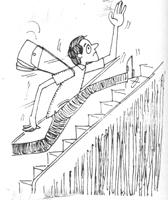The 7 additional steps take 10.5 seconds. Therefore, each step takes 1.5 seconds. Total steps in escalator = 8 + 31.5 / 1.5 = 29 or Total steps in escalator = 15 + 21.0 / 1.5 = 29.

If Rajesh did not run up any steps at all, he would reach the top of the escalator in 43.5 seconds (i.e., 29 steps x 1.5 seconds/step). Alternative solution through Equation:
Let the total number of steps in the escalator be x. The escalator moves at a constant speed given by
Speed of escalator = (x - 8)/31.5 = (x – 15)/21.0
Then: x = (15 x 31.5 – 8 x 21.0) / (31.5 – 21.0) = 29.
Now, speed of escalator = (29 - 8)/31.5 = (29 – 15)/21.0 = 1/1.5 steps/second. Time to reach top = Total steps / speed = 43.5 seconds.

#### Chess Board

You have two chess boards B1 and B2. There are 64 coins on first chess board B1, of which 16 are Tails, all the others Heads.

All are arranged randomly on squares. You are blindfolded and cannot tell the upside by ‘feeling’ the coins either. You can transfer as many coins as you wish from the chess board B2, and you may choose to flip any coin you transfer, if you want to. The goal is to have an equal number of Tails on both boards. How do you achieve that? Note that you can only transfer back once you put anything on B2!!

Ans: Select any 16 coins from B1. Let’s assume x of these are all tails and other 16-X are heads. Flip them all over and put them on B2. So now we have left on B1: 16-X tails and heads 32+X heads and on B2: 16-X tails and X heads.Thus we have an equal number of tails on both boards.

#### How big is the largest square table?

How big is the largest square table that you can completely cover with three 1 meter square table cloths? No cutting or folding allowed!!(You can express the answer as root of something)

Ans:See the given figure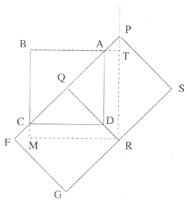Let ABCD, PQRS and QFGR represent three 1 meter square table clothes. It is clear from the figure that such an arrangement will cover a square BTRM [dashed line]
We know that BA=IM
BT=BA+AT = 1+ AT Where AT is unknown. Extend the side RT such that it hits P.(dotted line).Now it is clear that ATP and ADQ are similar triangles.

So,
AT = AP

=) AT= (AP) AQ= (1-√2) √2
2
=) AT=√2 - 1 = √2-1
2 2 2
Side of the largest square=
BA+AT=1+ √2-1 = 1+√2
2 2
[Which is approximately1. 207m?]

#### Multiplication with 0

Why is it when I have 0 divided by 2, I will get an answer 0, but when I have it the other way round 2 divided by 0, I get an error with my electronic calculator?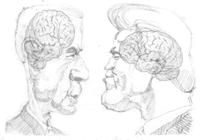We have 2+3 = 5. Therefore we have 5 – 2 = 3 or 5 – 3 = 2. That is to say, subtraction is the inverse operation of addition. In the same way, we have 2 × 3 = 6. Therefore we have 6 / 2 = 3 or 6 / 3 = 2. That is to say, division is the inverse operation of multiplication. Since 2 × 0 = 0 and 3 × 0 = 0, we have a problem here.

We cannot find a number whose multiplication with 0 is 2. So we cannot find an answer for 2 divided by 0. Whatever I say would not be correct because its multiplication with 0 would not give us 2. If something we cannot do an easy way out is not to do it. Henceforth your calculator also shows an error.

#### How many times in a day (24 hours) do the hands form right angles in the clock?

At 3 o’clock and 9 o’clock, the hour hand and minute hand of a clock form a right angle. In all, how many times in a day (24 hours) do the hands form right angles?
Answer: Starting from 12:00, the hands from right angles at approximately 12:17, 12:49, 1:22, 1:55….or twice an hour. There are two exceptions. Between 2:00 and 3:00, the hands form right angles only once, around 2:27. Similarly between 8:00 and 9:00, the hands from right angles only once, around 8:27. This means that the hands from right angles 22 times in 12 hours, or 44 times in 24 hours.

How fast does the water in the canal flow?

A swimmer jumps from a bridge over a canal and swims 1 kilometer stream up. After that first kilometer he passes a floating cork. He continues swimming for half an hour and then turns around and swims back to the bridge. The swimmer and the cork arrive at the bridge at the same time. The swimmer has been swimming with contest speed. How fast does the water in the canal flow?

Answer: It is obvious that the cork does not move relatively to the water (i.e. has the same speed as the water).So if the swimmer is swimming away from cork for half an hour (up stream), it will take him another half hour to swim back to the cork again. So in that on hour time, the cork has floated from 1 kilometer up stream to the bridge. Conclusion: The water in the canal flows at a speed of 1 km/h.

#### Rectangular to square of dimension

You are given a rectangular piece of cloth of dimensions 16 cm by 9 cm. How will you cut the cloth into two so that when you join it up, you have a square of dimensions 12 cm by 12 cm?

Solution:
See the picture. Note that this can be done given any rectangle of dimensions
m2 and (m + 1) 2, with one cut, we can make two pieces and join them to make a square whose side is m(m+1).
A more general version of this question, dealing with more general shapes (polyhedral) and volumes, was posed by David Hilbert in his famous 1900 speech of the congress of Mathematicians, Paris.

This problem, the third in Hilbert’s list of 23 problems, was in fact the first to be solved. The study of geometric dissections, where one changes the shapes of geometric objects while preserving area or volume, is an exciting branch of mathematics.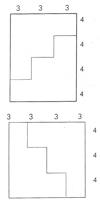#### You have 3 large bananas, and a knife. How will you make 14 pieces from them, using only 3 cuts?

Solution

First line up the three bananas and cut them in the middle in one shot. You have six pieces on hand, line them, and in one cut, make them into 12 pieces.
Take two pieces out of these twelve, and in one more cut, halve them to get 14 pieces as required.

#### How many lockers will be open at the end?

1. There are 1000 lockers in a high school with 1000 students. The problem begins with the first student opening all 1000 lockers; next the second student closes lockers 2,4,6,8,10 and so on to locker 1000; the third student changes the state (opens lockers closed, closes lockers open) on lockers 3,6,9,12,15 and so on; the fourth student changes the state of lockers 4,8,12,16 and so on. This goes on until every student has had a turn.

How many lockers will be open at the end?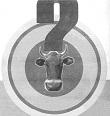Answer: The correct answer is 31 locks are open how we do solve this question? One thing we can do is to let the first 10 students to go their open/shut operation with the locks. The students who come after them are not going to touch lockers 1-10 so we can see which ones in that first batch are still open and try to guess the pattern.

When we do that we find that locks 1, 4 and 9 are open and the others are closed. Now, that isn’t much to go on so maybe you could let the next 10 students go do their operation. When the first 20 lockers are through being touched, and we find that lockers 1, 4, 9 and 16 are the only ones in the first 20 that are still open. So what is the pattern?

Let’s take any add locker, like 48 for example. It gets its state altered once for every student whose number in line is an exact divisor of 48. The chart shows this clearly:

Student No: leaves locker 48

1 open

2 shut

3 open

4 shut

5 open

8 shut

12 open

16 shut

24 open

48 shut

Notice that 48 has an even number (ten)of divisors namely 1,2,3,4,6,8,12,16,24,48. So the locker goes open-shut-open-shut……and ends up shut. Any locker number that has an even number of divisors will end up shut.

Which numbers have an odd number of divisors? That’s the answer to this problem. Just to help you along here are the locker numbers up to 100 that are left open:

1,4,9,16,25,36,49,64,81,100.

If you can describe these numbers in a different way from “having an odd number of divisors,” there is one more interesting pattern hidden in this series. Notice that the above numbers make n square series. Thus there are 31 of the 1000 lockers still open.

#### How many shake hands?

There are 40 people shake hands. So each person shakes 39 hands. The first person shakes 39 hands, the second person shakes 38 hands, and the third person shakes 37 hands. And so on. The total number of handshakes is

39+38+37+36+…..+3+2+1

To find this total without having to add all these numbers together, notice the following:

The average of the first and last numbers is 20: (39 + 1) / 2 = 20.

The average of the second and next-to-last number is 20: (38+2)/2 = 20.

If you think about it, you should be able to see that all the numbers can be paired in groups of two whose average is 20. You will have 39 numbers 1 to 39 whose average is 20 so what is the sum of all those numbers? It is just the number of numbers multiplied by the average of the numbers: 39 * 20=780.

#### How many phone numbers can be made?

How many phone numbers can be made under the following conditions: (First digit cannot be 0 or 1 because you’ll get the operator or long distance?

a) The first two digits are 3 followed by 6

b) The third digit is even

c) The fourth digit is greater than 5

d) The fifth and seventh digits are odd

e) The sixth digit is 2. One way to approach this problem is to count and see how many choices we have for each digit and then multiply them together. Assuming that you are talking about 7 digit phone numbers we note the following:

1. For the first digit we have only one choice we must choose a 3.
2. For the second digit, we have only one choice we must choose a 6.
3. For the third digit, we have five choices we can choose 0, 2, 4, 6 or 8
4. For the fourth digit we have four choices we can choose 6, 7, 8 or 9.
5. For the fifth digit, we have five choices we can choose 1,3,5,7 or 9
6. For the sixth digit, we have one choice we must choose 2,
7. For the seventh digit we have five choices we can choose 1, 3, 5, 7 or 9

All the possibilities are multiplied to get total phone numbers, 1 x 1 x 5 x 4 x 1 x 5 = 500 phone numbers.

Source: Portal Content team

© 2006–2019 C–DAC.All content appearing on the vikaspedia portal is through collaborative effort of vikaspedia and its partners.We encourage you to use and share the content in a respectful and fair manner. Please leave all source links intact and adhere to applicable copyright and intellectual property guidelines and laws.
English to Hindi Transliterate